Plastic rectangle and Padovan sequence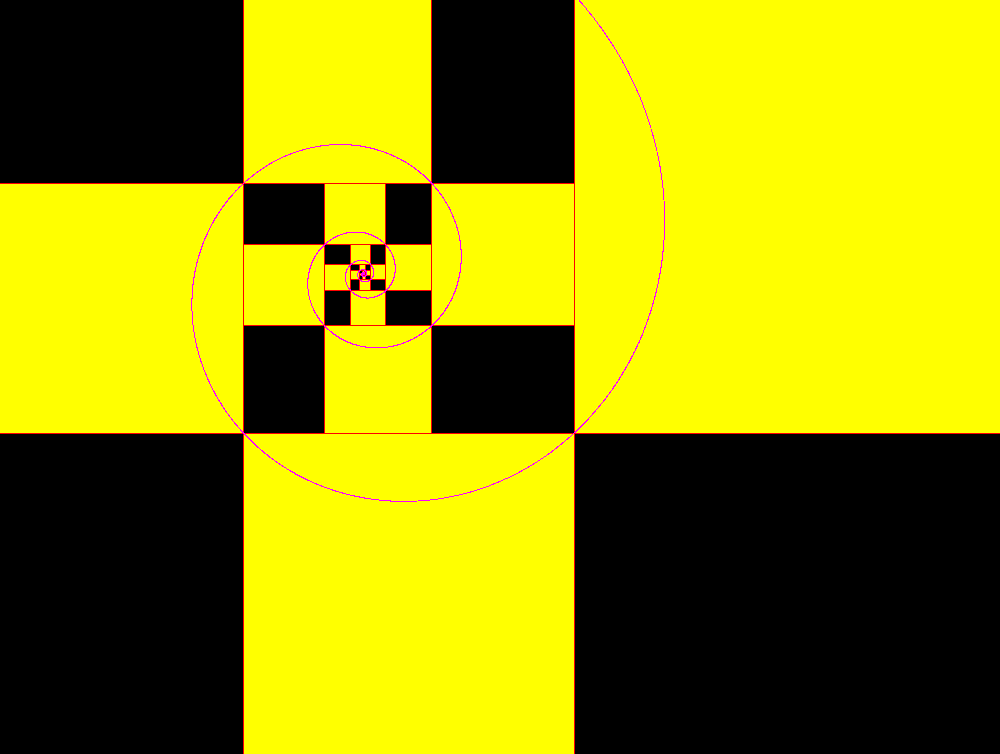It is observed that the side of a square equals the sum of the two not immediately preceding. For this you can tend to the plastic rectangle building rectangles with square in the following sequence: 1, 1, 1, 2, 2, 3, 4, 5, 7, 9, 12, 16, 21, 28, 37, 49, 65, 86, 114, 151, 200, 265, 351, 465, 616, 816, 1081,... that it is the famous Padovan numbers Ratio of two consecutive numbers of the sequence tends to the relationship between major and minor side of the golden rectangle :: P=1.324717957... plastic number       one of the solutions of the following equation: r3-r-1=0 (1/P=0.7548776662...one of the solutions of the following equation: r3+r2-1=0)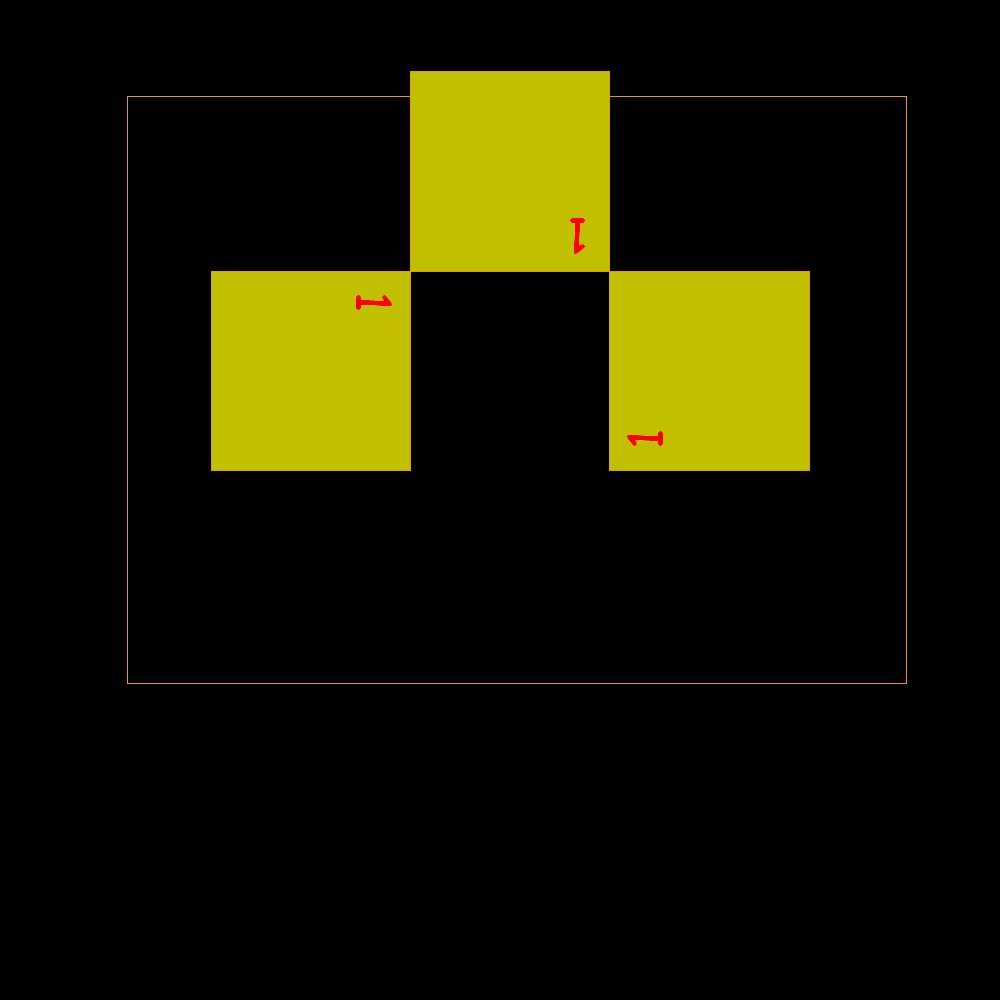Start of rectangles identified by square building with sides according to the Padovan sequence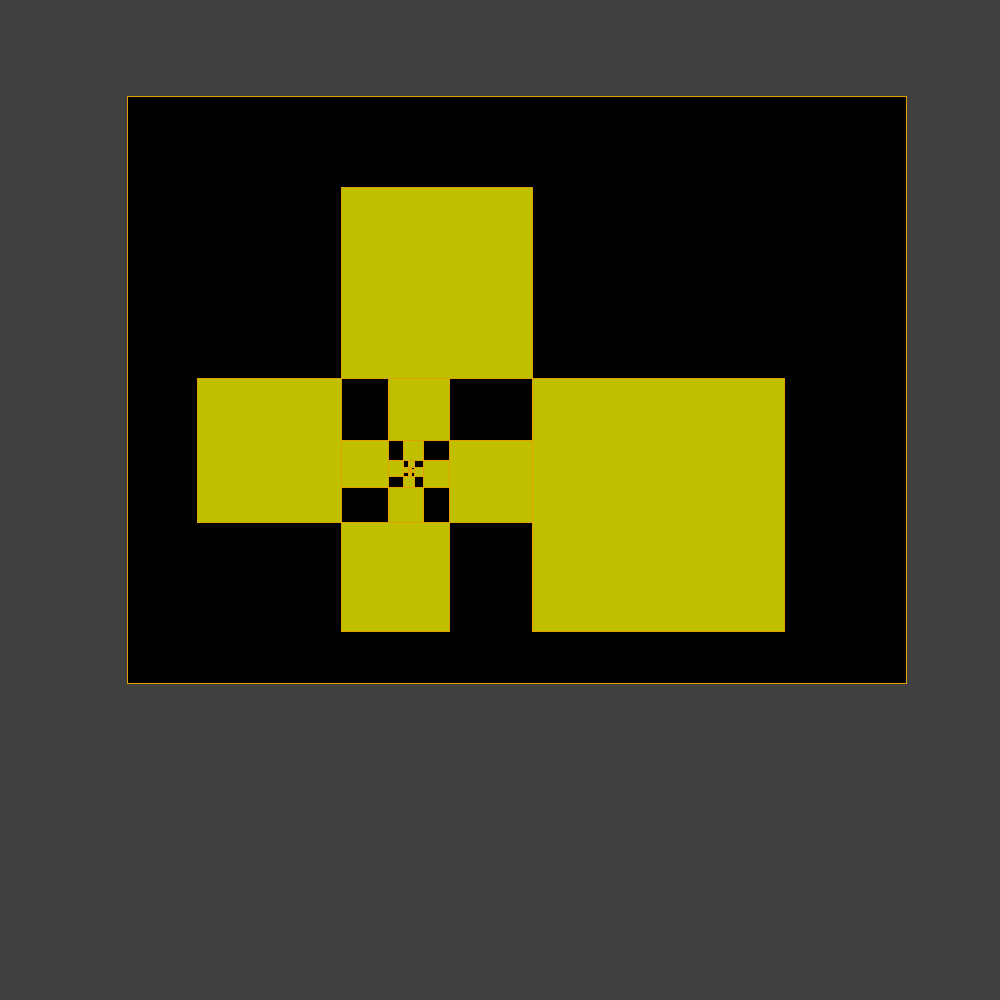Shortly after the result is confused with the plastic rectangle to which it tends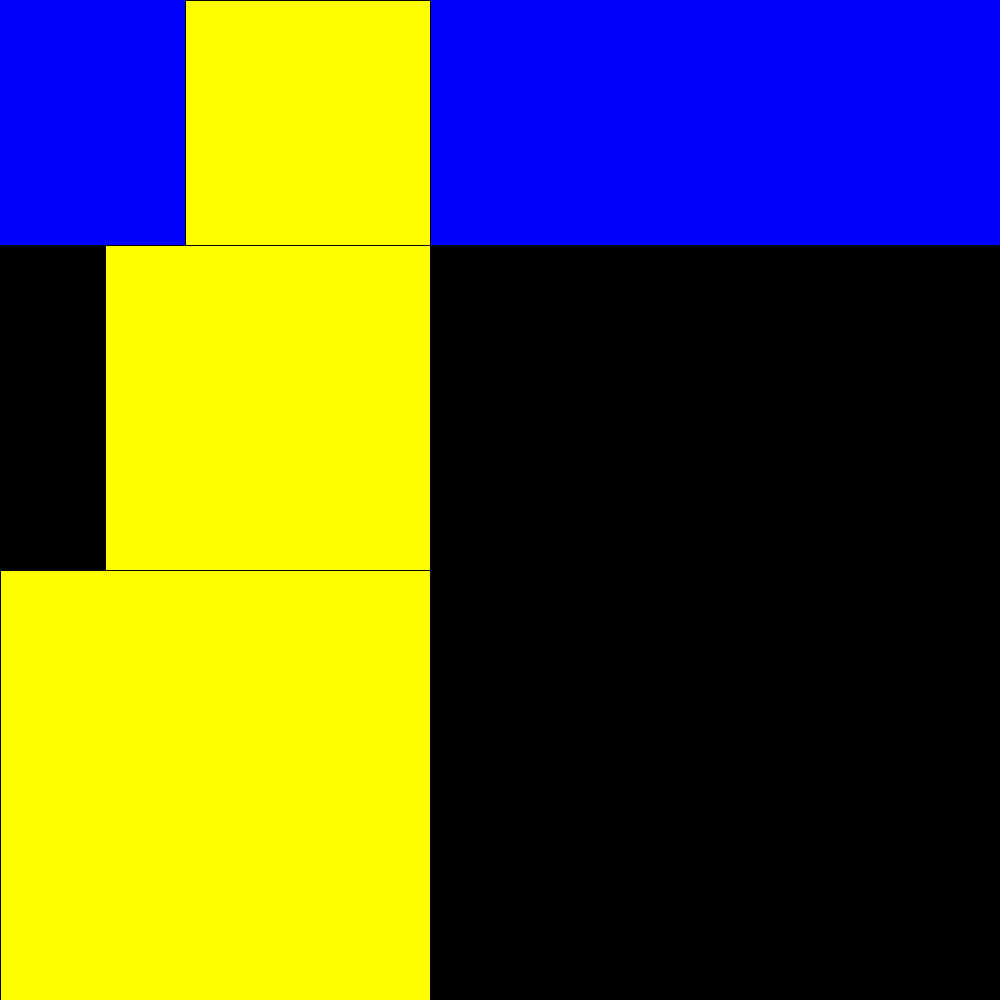packing and unpacking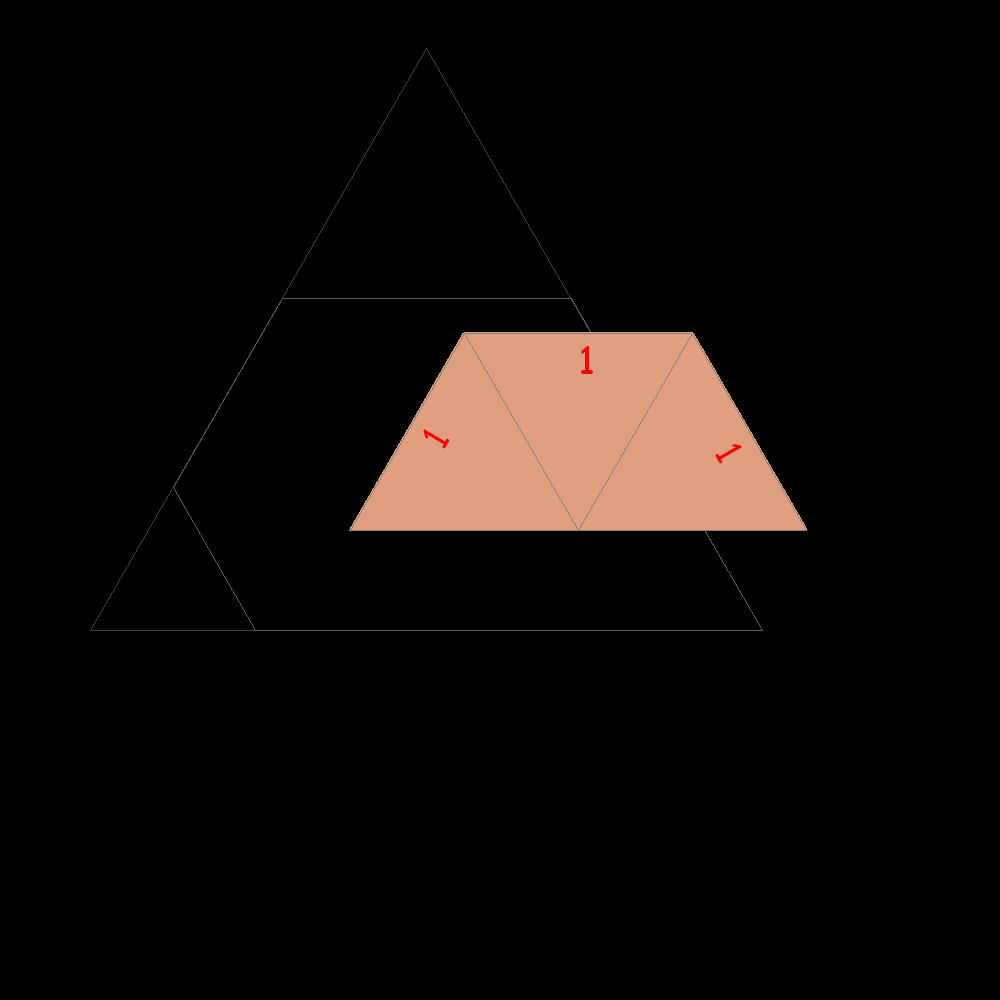Start of construction of trapezes with equilateral triangles according with the  Padovan sequenceShortly after the result is confused with the plastic trapeze to which it tends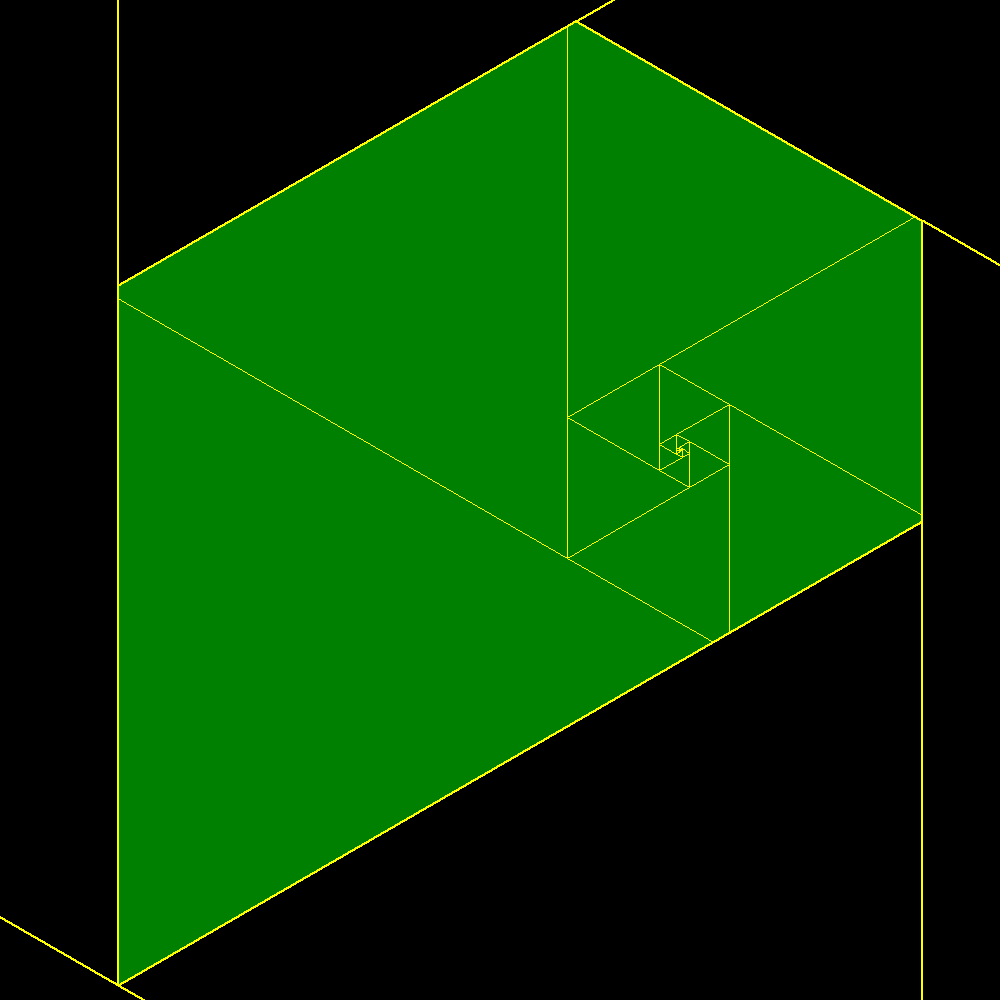Plastic trapeze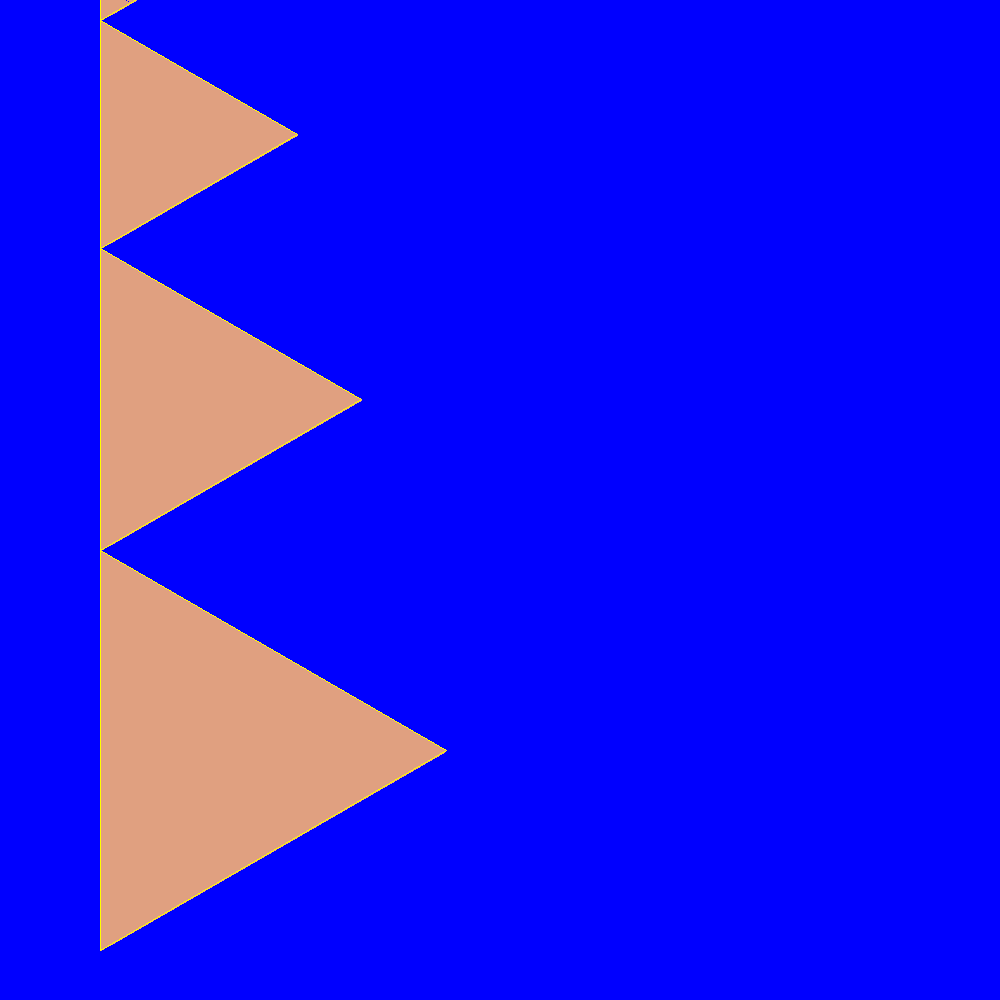packing and unpacking